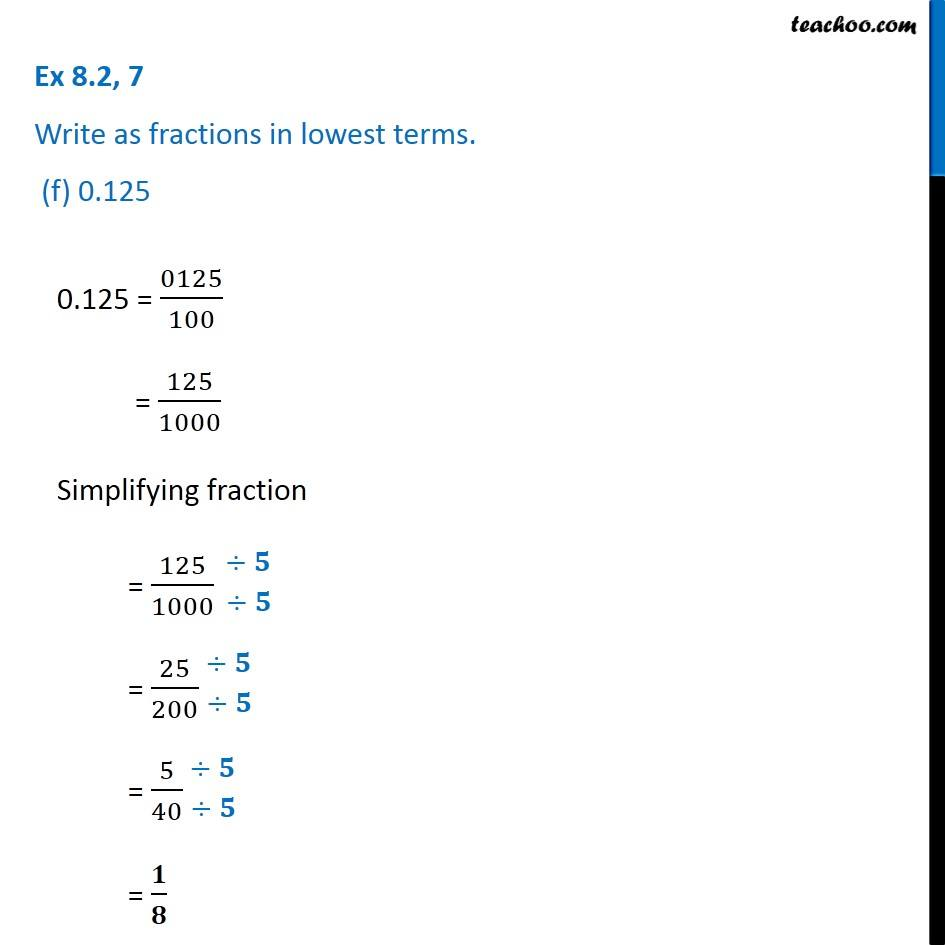1. Chapter 8 Class 6 Decimals
2. Serial order wise
3. Ex 8.2

Transcript

Ex 8.2, 7 Write as fractions in lowest terms. (f) 0.125 0.125 = 0125/100 = 125/1000 Simplifying fraction = 125/1000 = 25/200 = 5/40 = 𝟏/𝟖

Ex 8.2

Chapter 8 Class 6 Decimals
Serial order wise